# Calculus 1 : Graphing Functions

## Example Questions

1 2 20 21 22 23 24 25 26 28 Next →

### Example Question #26 : How To Find Slope By Graphing Functions

Find the slope of the line tangent to the curve of g(x) when x is equal to 5.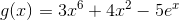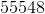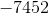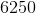Explanation:

Find the slope of the line tangent to the curve of g(x) when x is equal to 5.To find the slope of a tangent line, first find the derivative of the beginning functions:Will become: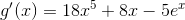Next, simply plug in 5 everywhere we have an x and solve.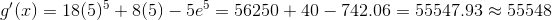### Example Question #27 : How To Find Slope By Graphing Functions

Find the slope of the line tangent to h(x), when.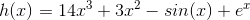Explanation:

Find the slope of the line tangent to h(x), when.To find the slope of a tangent line, we need to find the derivative of our function:

Begin by recalling the rule for polynomial derivatives, derivative of sine, and derivative of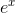Polynomials derivatives are found by decreasing our exponent by one, and then dividing by that number.

The derivative of sine is cosine

The derivative ofisSo with that in mind, let's find h'(x)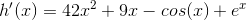Next, we need to find h'(0), so plug in 0 for x and simplify: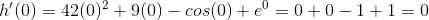So our slope is 0 when x=0.

### Example Question #28 : How To Find Slope By Graphing Functions

Find the slope of the line tangent to f(x) when x is -5.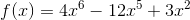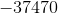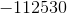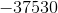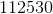Explanation:

Find the slope of the line tangent to f(x) when x is -5.To find the slope of a tangent line, first find the derivative. Then, plug in the given point. Recall the power rule of derivatives. (Multiply each term by its exponent, then subtract one from the exponent)Becomes: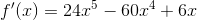Next, plug in -5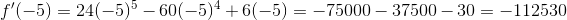In doing so, we arrive at -112530, a very steep slope indeed!

### Example Question #271 : Graphing Functions

If the equation for a graph given is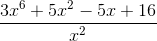, find the slope of a line tangent to this graph at.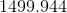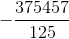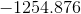None of the above.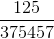Explanation:

In order to find the slope, you take derivative of the graph equation.  Then by plugging in anyvalue, you can find the slope of the graph.

In order to take the derivative of equation, the power rule must be applied,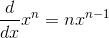.  You must also apply the quotient rule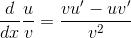.

Taking the derivative of the graph equation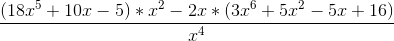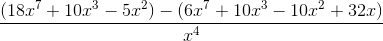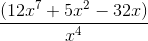937,500+125-160

Plugging in, you find the slope to be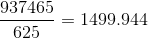.

1 2 20 21 22 23 24 25 26 28 Next →

### All Calculus 1 Resources